SSAT Upper Level Math : How to find the circumference of a circle

Example Questions

1 3 Next →

Example Question #21 : How To Find The Circumference Of A Circle

Find the circumference of a circle with a diameter of 6.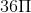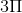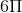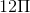Explanation:

There are two possible formulas for finding the circumference of a circle. They are as follows: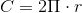And: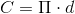Where C is circumference, r is radius, and d is diameter.

For this problem, we are given the diameter is 6, meaning we can plug our numbers into the second equation. Since pi is an irrational constant, it is okay to leave the answer in terms of pi.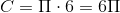Example Question #21 : How To Find The Circumference Of A Circle

Find the circumference of a circle with a diameter of 2.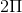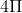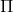Explanation:

There are two possible formulas for finding the circumference of a circle. They are as follows:And:Where C is circumference, r is radius, and d is diameter.

For this problem, we are given the diameter is 2, meaning we can plug our numbers into the second equation. Since pi is an irrational constant, it is okay to leave the answer in terms of pi.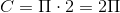Example Question #23 : How To Find The Circumference Of A Circle

Find the circumference of a circle with a radius of 12.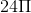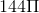Explanation:

There are two possible formulas for finding the circumference of a circle. They are as follows:And:Where C is circumference, r is radius, and d is diameter.

For this problem, we are given the radius is 12, meaning we can plug our numbers into the first equation. Since pi is an irrational constant, it is okay to leave the answer in terms of pi.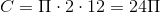Example Question #24 : How To Find The Circumference Of A Circle

Find the circumference of a circle with a radius of 13.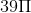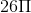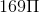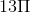Explanation:

There are two possible formulas for finding the circumference of a circle. They are as follows:And:Where C is circumference, r is radius, and d is diameter.

For this problem, we are given the radius is 13, meaning we can plug our numbers into the first equation. Since pi is an irrational constant, it is okay to leave the answer in terms of pi.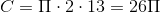Example Question #25 : How To Find The Circumference Of A Circle

Find the circumference of a circle with a radius of 27.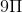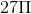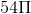Explanation:

There are two possible formulas for finding the circumference of a circle. They are as follows:And:Where C is circumference, r is radius, and d is diameter.

For this problem, we are given the radius is 27, meaning we can plug our numbers into the first equation. Since pi is an irrational constant, it is okay to leave the answer in terms of pi.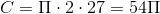1 3 Next →